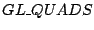Next: Source Code Up: How To Implement a Previous: Integrate Newton's Equations

# Visualization

For OpenGL visualization of the two dimensional soft body I usedtype which gave me simply way to draw filled body. Below a complete GLUT callback procedure which draw a body in a GLUT window is presented:

```void Draw(void)
{
int i;
glClearColor(1,1,1,0);
glClear(GL_COLOR_BUFFER_BIT);

glBegin(GL_QUADS);
for(i = 1 ; i <= NUMS-1 ; i++)
{
glColor3f(1,0.4,0.4);
glVertex2f(myPoints[ mySprings[i].i ].x,
myPoints[ mySprings[i].i ].y);
glVertex2f(myPoints[ mySprings[i].j ].x,
myPoints[ mySprings[i].j ].y);

glVertex2f(myPoints[ NUMP -
mySprings[i].i + 1].x,
myPoints[ NUMP -
mySprings[i].i + 1].y);
glVertex2f(myPoints[ NUMP -
mySprings[i].j + 1].x,
myPoints[ NUMP -
mySprings[i].j + 1].y);
}
glEnd();

glutSwapBuffers();
}
```

As we see in above code we simply draw QUADS (i,j,NUMP-i+1,NUMP-i+1), it is done as simply as possible and of course some nice features can be added here easly (normal/velocity vectors drawing, color differences while pressure changes etc.).

Maciej Matyka 2004-03-30